# Genuary 19

example p5.js javascript images genuary

``````let img;

}

function setup() {
createCanvas(400, 400);
img.resize(width, height);
noSmooth();
image(img, 0, 0);
}

function draw() {
const y = frameCount % height;
const range = getPixelRange(y);

for (let x = 0; x < width; x++) {
const leftX = constrain(x - range, 0, width);
const rightX = constrain(x + range, 0, width);
let sampleX = random(leftX, rightX);

const topY = constrain(y - range, 0, height);
const bottomY = constrain(y + range, 0, height);
let sampleY = random(topY, bottomY);

const pixelColor = img.get(sampleX, sampleY);

stroke(pixelColor);
point(x, y);
}
}

function getPixelRange(y) {
return map(pow(y, 3),
0, pow(height, 3),
0, 50);
}
``````

This sketch increases the randomness of an image along the Y axis.

I created this for the 19th day of Genuary which had a prompt of “Increase the randomness along the Y-axis.”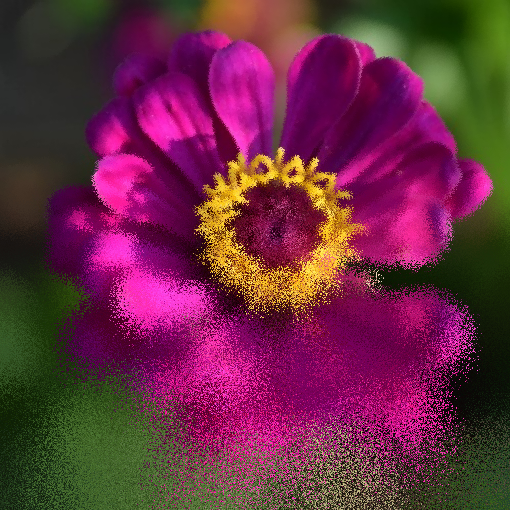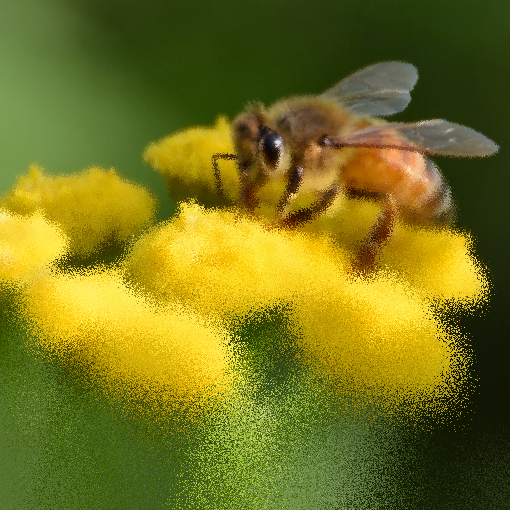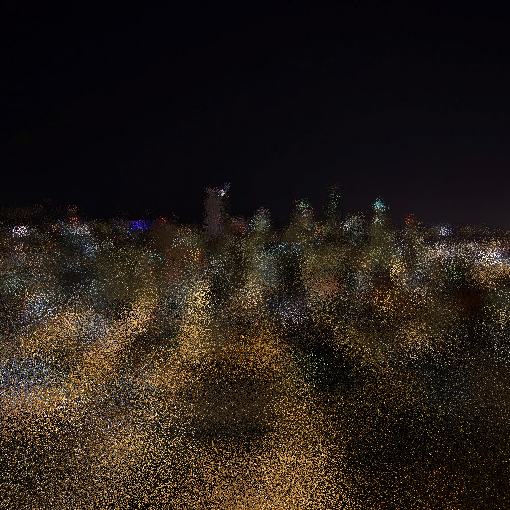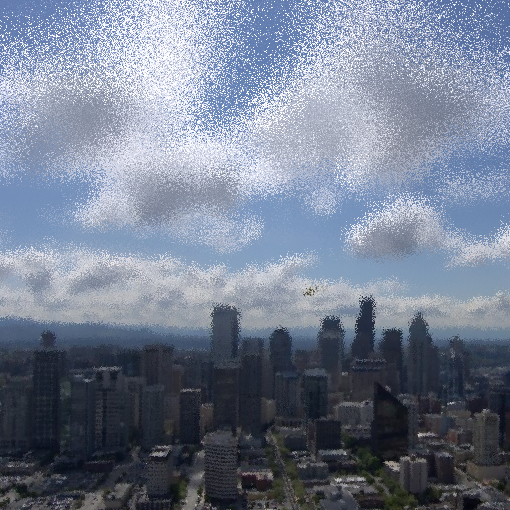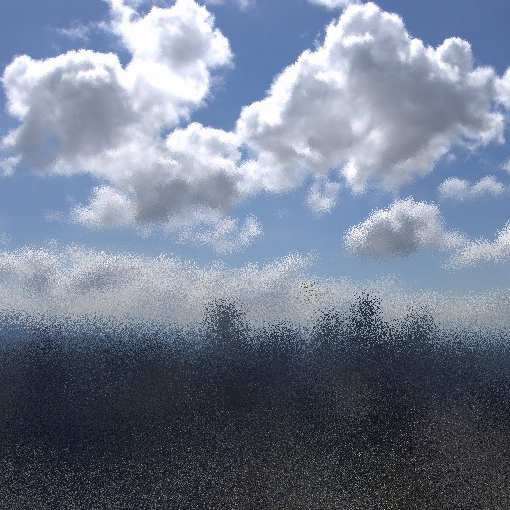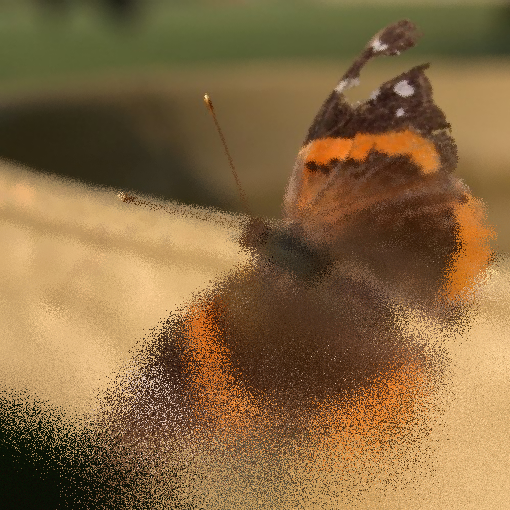# Remix Ideas

• Apply this filter to different images.
• Modify the `getPixelRange()` function to create different effects.
• Instead of picking a random pixel, add randomness to the color of the pixel.